ISSN ONLINE(2319-8753)PRINT(2347-6710)

All submissions of the EM system will be redirected to Online Manuscript Submission System. Authors are requested to submit articles directly to Online Manuscript Submission System of respective journal.

# Implementation of Crosstalk-Resistant Adaptive Decorrelator using Labview

 Katta Prathyusha1, M.S.Pradeep Kumar Patnaik2 P.G. Student, Dept. of Electronics and Instrumentation Engineering, GITAM University, Visakhapatnam, A.P, India Asst. Professor, Dept. of Electronics and Instrumentation Engineering, GITAM University, Visakhapatnam, A.P, India Related article at Pubmed, Scholar Google

Visit for more related articles at International Journal of Innovative Research in Science, Engineering and Technology

## Abstract

In this paper we designed an adaptive decorrelator which is based on two cross coupled least-mean square algorithms. This decorrelator is used to remove the crosstalk which may occur during echo in telephone networks, while two or more persons talking at the same time. This paper deals with separation of two sound (voice) signals which are mixed in real environment. This separation is done by decorrelating cross coupling transfer functions which link the two sound signals. The decorrelator is implemented using LabVIEW, since it is convenient for implementing such algorithms. Here LMS algorithm starts with weight vector zero and iteration continues till the error is minimized. This work has application in smart phones, hearing aid systems and speech recognition systems.

### Keywords

Adaptive Decorrelator, Cross Coupled LMS algorithm, LabVIEW,crosstalk.

### INTRODUCTION

Crosstalk is the phenomenon by which a signal transmitted on one circuit or channel creates an undesired effect in another circuit or channel. Crosstalk elimination is necessary to increase signal to noiseratio(SNR).Sometimes crosstalk can also be resulted because of undesired effect causedby coupling capacitance of the network.Hence elimination of crosstalk is one of the major and important tasks in many fields.
Here we are implementing adaptive filtering based on Cross Coupled LMS algorithm.LabVIEW has existed since the mid 1980âs and is usually associated with virtual Instrumentation.It is a graphical language indicated by „gâ which in turn indicates that it is a block diagram approach to programming.LabVIEW is a programming language which is complied and executed when the run button is pressed.
In this paper we are using NI LabVIEW 2014,a graphical programming language tool provided by National Instruments,which is used for simulation.There are many advantages of using LabVIEW,some of them are:
• Reduction in size of code
• Easy to understand and implement the code
• Provide better debugging capabilities
• Code execution is faster
ï· Intuitive working environment

### RELATED WORK

According to the various research papers, we are concerned with the different reduction techniques based onfiltering and FFT. Adaptive filtering is one of the most important field which explores crosstalk reduction using different algorithms like Least Mean Square(LMS), Fast Block Least Mean Square(FBLMS) and Fast Block Least Mean Square with Distributed Arithmetic(FBLMS with DA).Adaptive Noise Cancellation (ANC) applications use adaptive filters which are filters whose coefficients adjust automatically according to certain criteria. Some fundamental criteria are least mean squares (LMS), normalized LMS, and recursive least squares (RLS). Researchers and engineers use adaptive filters to perform noise cancellation, echo cancellation, system identification, and other applications.
Many scientists have worked on ANC to produce practical applications. The following studies are some examples that show ANC's importance in noise cancellation:• C.J. James shows that multireference ANC can be used to enhance transient non-stationarities in electroencephalograms (EEG).
• Muhammad Afzal claims that ANC can help to better evaluate a gas pipeline system.
• GeorgiIliev indicates that voice and speech recognition can use ANC.
The following figure shows typical Noise Cancellation System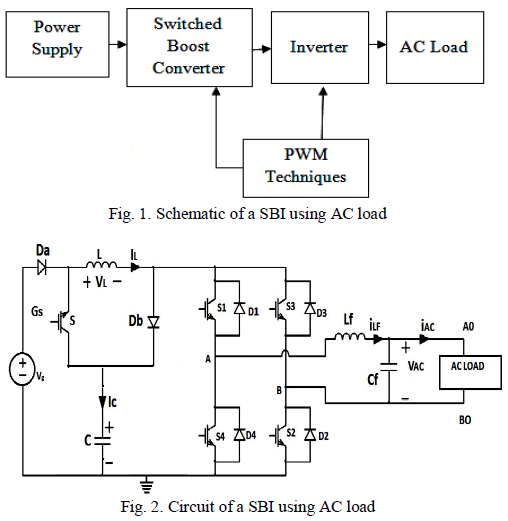Fig.1 Typical Adaptive Noise Cancellation System
Where s(n) is the pilot's speech you need to acquire, v(n), v1(n), andv2(n) represent the jet engine noise, y(n) is the output signal, e(n) is the resulting signal. The communication between a pilot inside a jet craft and a ground control tower is usually polluted with jet engine noise. In Figure 1, you cannot acquire s(n) or v1(n) directly. We can acquire s(n)+v1(n). To remove v1(n) from s(n)+v1(n), you can use an adaptive filter. Wehave to use a sensor to acquire only the jet engine noise v2(n) and send this signal to the adaptive filter. If s(n) is uncorrelated with the jet engine noise v(n), and if both v1(n) and v2(n) are highly correlated with v(n), the noise cancellation system can estimate the jet engine noise v1(n) by adjusting the coefficients of the adaptive filter iteratively. When the output signal y(n) becomes close to v1(n), the system can then remove the engine noise. In Figure 1, e(n) denotes the resulting signal that is close to s(n).

### THEORITICAL ANALYSIS

We have two signals rk 1,rk 2 which are mixed in the natural acoustics occurred in a room.Figure2 shows this.The aim of this paper is to separate these two signals using blind source separation.Here blind source separation indicates that there is no prior information about these signals,all that we know is there are two random signals.Then let us consider the received signals as sk 1,sk 2.Hence we have a 2x2 coupling matrix of FIR transfer functions H11.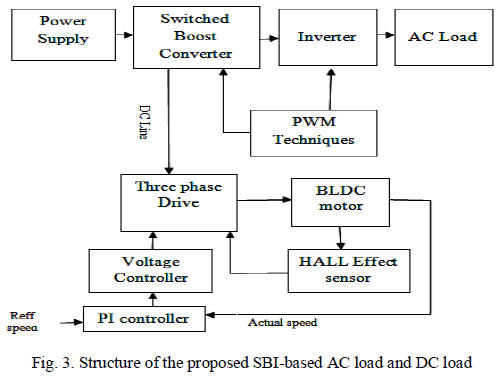Fig.2Acoustic mixing of two signals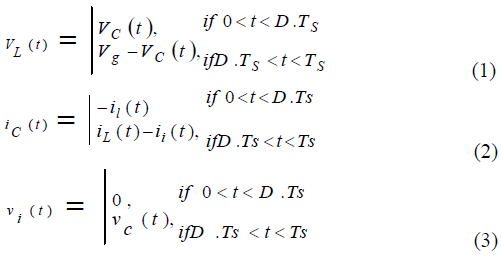The two decorrelated random signals are tk i.Here we make g12(0)=g21(0)=0 for strict causality of two polynomials.μ1,μ2 are the step sizes of the individual LMS algorithms.Usually μ1=μ2=μ, since the algorithm is to be symmetric.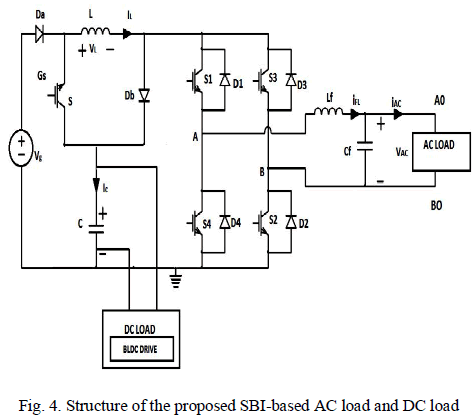Fig.3Two Input Decorrelator
The above algorithm results in biased estimates and does not produce optimal estimates as in the case of general LMS algorithm, though thisalgorithm produces excellent performance in real time environment.

### PROCESS

Two sound signals are recorded simultaneously in real environment and stored as an audio file for further processing.The signals are separated using Cross Coupled Least Mean Square(LMS) algorithm.For „nâ number of weights the algorithm requires approximately 2n multiplications and 2n additions where as ordinary LMS algorithm requires „nâ number of multiplications and additions.The advantage of using Cross Coupled LMS is its ability to split the overall filter of order n into two filters of size n/2.The algorithm provides two outputs and these two form the inputs for the next stage.
Here this algorithm is implemented using NI LabVIEW 2014.The algorithm is implemented as the sub-vi,which includes the concept of pipelining.Pipelining here indicates the use of feedback nodes and shift registers,this allows items to execute in parallel instead of serial execution.The general computation of LMS algorithm is split into parallel loops without affecting stability of system.This is faster when compared with the general LMS.Figure4 illustrates Cross Coupled LMS algorithm in LabVIEW.Fig.4Portion of Cross Coupled LMS
The above figure shows that the whole code is placed in for loop which is executed of n number of iterations.The above program takes two array inputs,no of weights to be calculated andstep size of the individual LMS algorithms.The registers are used to store the results of the current iteration and pass them to the next iteration. The above code uses different array functions,numeric functions,structures etc.,in order to implement Cross Coupled LMS. The final output of thealgorithm is two separate signals and their corresponding weight vectors.
The following Figure5 shows the block diagram of the normalized sub-vi. The signals are normalized to reduce the complexity in calculations.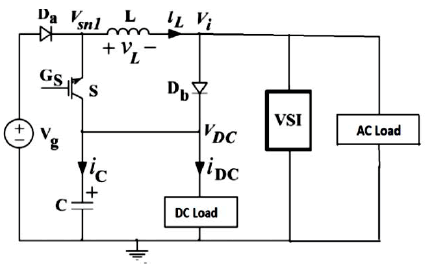Fig.5 Normalization of signal

### IMPLEMENTATION

The above mentioned sub-viâs are used in the main program in order to implement decorrelator.The main program uses different LabVIEW tools like file I/O functions i.e. “file dialog express vi” which is used to specify the path for the file or directory.We use this function to specify the path of the input audio file.This file is read using LabVIEW library function called “Snd read wavefile.vi”.After the signal is read it is split into two arrays using array functions and are converted to single precision floating point number format.Then these arrays are given as input to the Cross Coupled LMS algorithm(sub-vi).Then the output of the LMS algorithm will be two separate sound signals.
These sound signals are then written individually on some output audio file using file I/O functions and LabVIEW library function called “Snd write wavefile.vi”.Figure6and Figure7illustrates above discussion.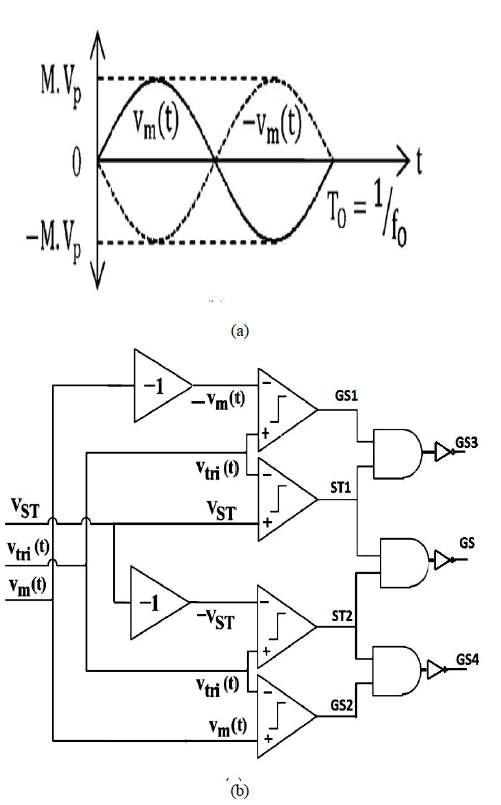Fig.6 Reading input audio signal and applying cross coupled LMS algorithm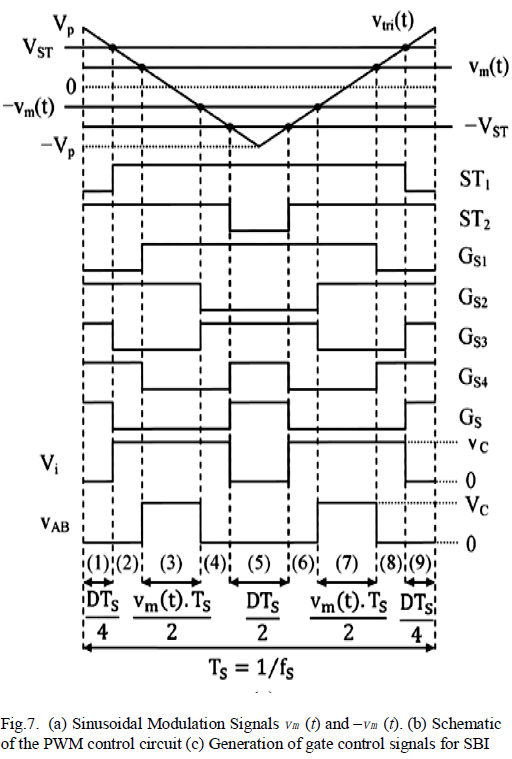Fig.7 writing output into audio file

### RESULTS

The size of the filter and step size of the LMS algorithm can be given in the front panel of the main program. Figure8 and Figure9 shows the input signals that are applied to the Cross Coupled LMS algorithm.Here signal1 is mixed with crosstalk of signal2 where as signal2 is mixed with crosstalk of signal1.### CONCLUSION

Hence we have designed an adaptive decorrelator which is used to separate two sound signals which are recorded simultaneously,by reducing the crosstalk between them.The LMS algorithm has been implemented using the graphical programminglanguage(LabVIEW).It has been shown how adaptive signal processing can be implemented efficiently and obtain the better results than other programming methods.In this paper we successfully separated two sound signals by removing crosstalk between them.

### References

T.J.Moir,Adaptive Noise Cancellation using LabVIEW.

Johnson,G.W.,LabVIEW graphical programming,McGraw Hill series,NY,1994.

MonsonH.Hayes,Statistical Digital Signal Processing and Modelling,John Wiley &Sons,Inc.USA

Chugani,M,L,Samant,A.R and Cerna,M., LabVIEW Signal Processing, National Instruments Series, Prentice Hall,NJ,1998

Haykin,S., Adaptive Filter theory, Prentice Hall Englewood Cliffs,NJ,1986

 FPGA based crosstalk-resistant adaptive decorrelatorT.J.Moir School of Engineering AUT University